﻿ 二维溃坝的数值模拟研究
«上一篇文章快速检索 高级检索

 应用科技2018, Vol. 45Issue (5): 6-10, 15  DOI: 10.11991/yykj.2017120070

### 引用本文HU Jian, LIU Lichao. Numerical simulation of 2D dam-break flow[J]. Applied Science and Technology, 2018, 45(5), 6-10, 15. DOI: 10.11991/yykj.201712007.### 文章历史

Numerical simulation of 2D dam-break flow
HU Jian, LIU LichaoCollege of Shipbuilding Engineering, Harbin Engineering University, Harbin 150001, China
Abstract: In order to solve fluid movement and evolution of the free surface of liquid in the dam-break problem, this paper presents a numerical simulation on the simplified block water collapse. The simulation uses the Finite Volume Method (FVM) to discretize the fluid control equation, applies the first order upwind scheme and center difference scheme to the convective term and diffusive term of the equation respectively; solves the discrete equations of the coupling pressure and speed by IDEAL; captures and reconstructs the free surface of liquid by the Volume of Fluid (VOF) method and the procedural language for integrity constraints (PLIC) method; and transforms the surface tension in the two-phase flow problem by the continuous surface force (CSF) model. On this basis, the two-dimensional dam-breaking problem and the problem of dam-breaking with barrier in a finite space were simulated respectively, and the free surfaces of liquid at several moments were captured and compared with the experiment result, proving the accuracy of the methods mentioned above.
Keywords: 2-dimensional dam-break    obstacle    N-S equation    finite volume method    volume of fluid    procedural language for integrity constraints    IDEAL    continuous surface force

Deborah M. Greaves基于VOF方法，以自适应网格对方柱水的坍塌及带障碍物坍塌过程进行了模拟，并进行了试验对比。Abdolmaleki等分别基于VOF方法和FLUENT软件对二维溃坝问题进行了计算。Jaswant等基于一种显式中心迎风格式进行了一维和二维的溃坝模拟计算以及带障碍物溃坝的计算。Brufau等基于Delaunay非结构网格对二维浅水方程进行求解，对二维溃坝问题进行了模拟分析。

1 理论基础 1.1 控制方程

 $\frac{{\partial \rho }}{{\partial t }} + \nabla \cdot (\rho {{V}}) = 0$

 ${\bf {\nabla}} \cdot {{V}} = 0$

N-S方程有以下形式：

 $\frac{{\partial \left( {\rho {{V}}} \right)}}{{\partial t}} + \nabla \cdot \left( {\rho {{V}} \otimes {{V}}} \right) = \nabla \cdot \left( {\mu \nabla \otimes {{V}}} \right) - \nabla P + {{g}} + {{{f}}_\sigma } + {{S}}$ (1)

1.2 控制方程离散及求解

 $\sum\limits_{nf = 1}^n {{{{u}}_f} \cdot {{{A}}_f} = 0}$

 $\frac{{{{V}}_P^{t + \delta t} - {{V}}_P^t}}{{\delta t}}{V_P} + \sum\limits_{f = 1}^n {{\rho _f}{F_f}{{V}}_P^{t + \delta t}} = \sum\limits_{F = 1}^N {{\nu _f}{A_f} \cdot \left( {\nabla \otimes {{V}}} \right)_f^{t + \delta t} + {{{S}}_{{{{V}}_P}}}{V_P}}$

N-S方程的求解是通过IDEAL算法实现的，其全称为内部双迭代高效算法。IDEAL算法基于semi-implicit method for pressure-linked equations (SIMPLE)开发而成。它通过对压力方程进行2次内部迭代计算，几乎完全舍弃了SIMPLE算法的2个基本假设，速度和压力得到了充分耦合，大大提高了算法的收敛性。

1.3 VOF和PLIC方法

VOF方法是基于流体体积分数 $F$ 这一概念提出的。流体体积分数为标量函数，定义为控制体积内特征流体所占体积与控制体体积的比值。以水为例，若控制体内没有水， $F$ 为0；若控制体被水充满， $F$ 为1；若有 $0 < F < 1$ ，则表示该控制体内有自由液面存在

 \left\{\begin{aligned}& \rho = F{\rho _L} + (1 - F){\rho _G}\\& \mu = F{\mu _L} + (1 - F){\mu _G}\end{aligned}\right.

 $\frac{{\partial F}}{{\partial t}} + {{V}} \cdot \nabla F = 0$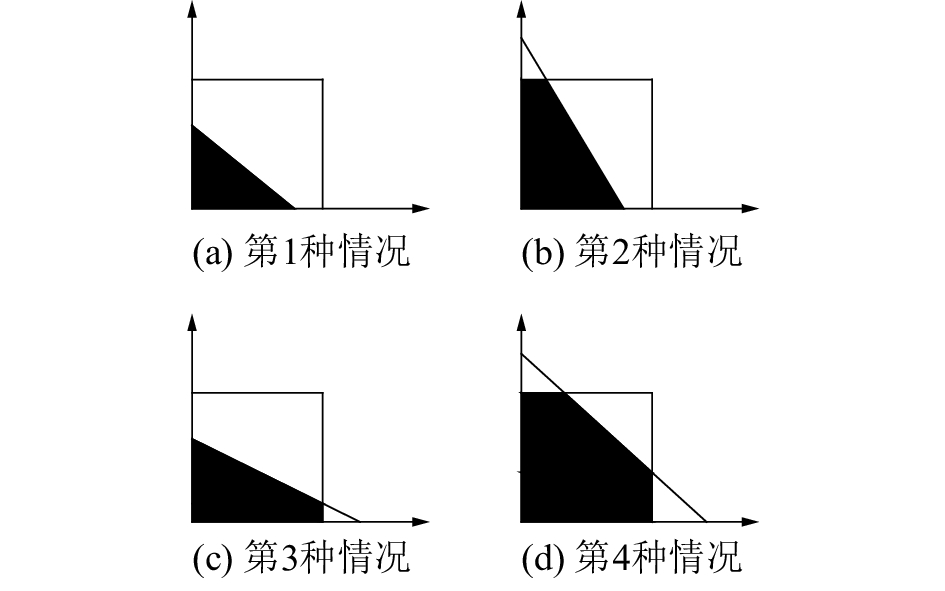Download: 图 1 PLIC界面重构的4种基本情况

1.4 表面张力的CSF模型

 $\Delta P = {P_I} - {P_O} = \sigma \kappa$

2 数值模型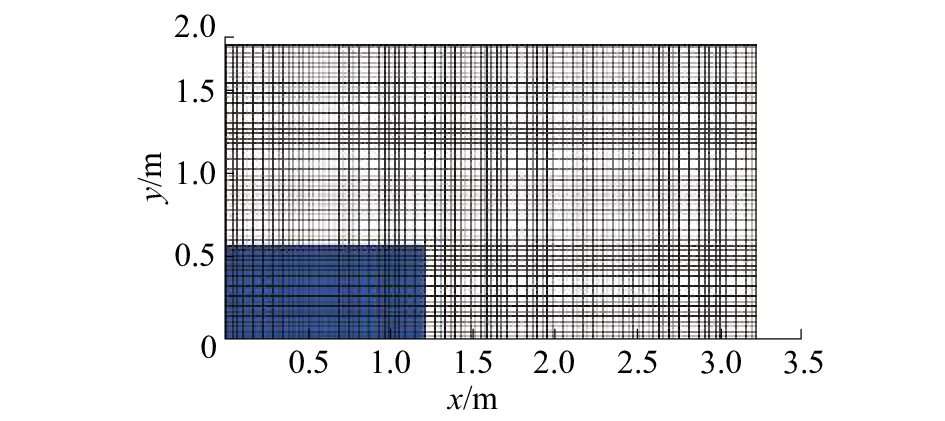Download: 图 2 二维溃坝模型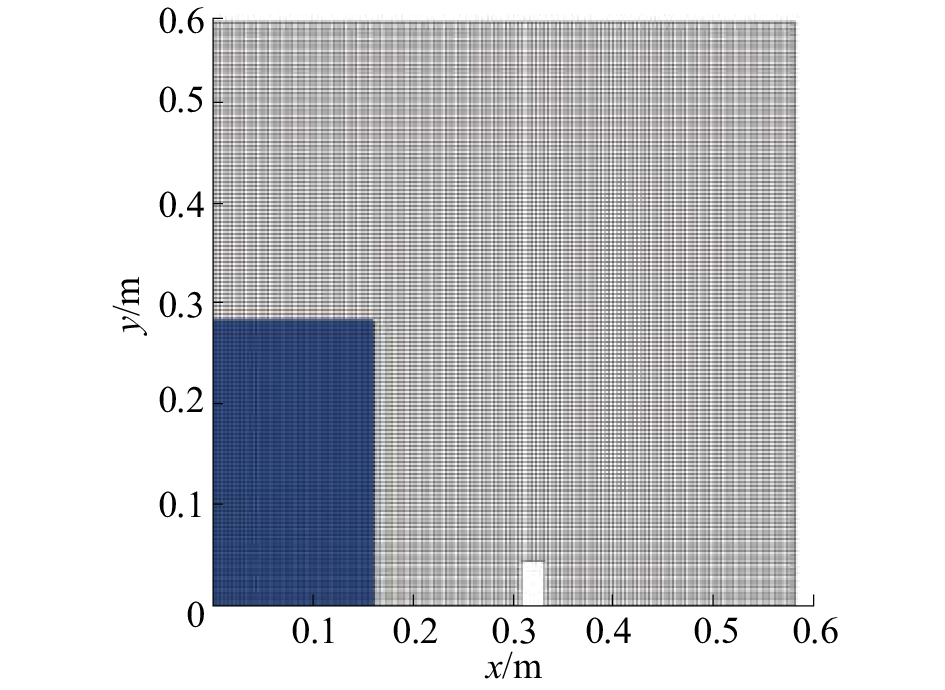Download: 图 3 带障碍物溃坝模型
3 数值结果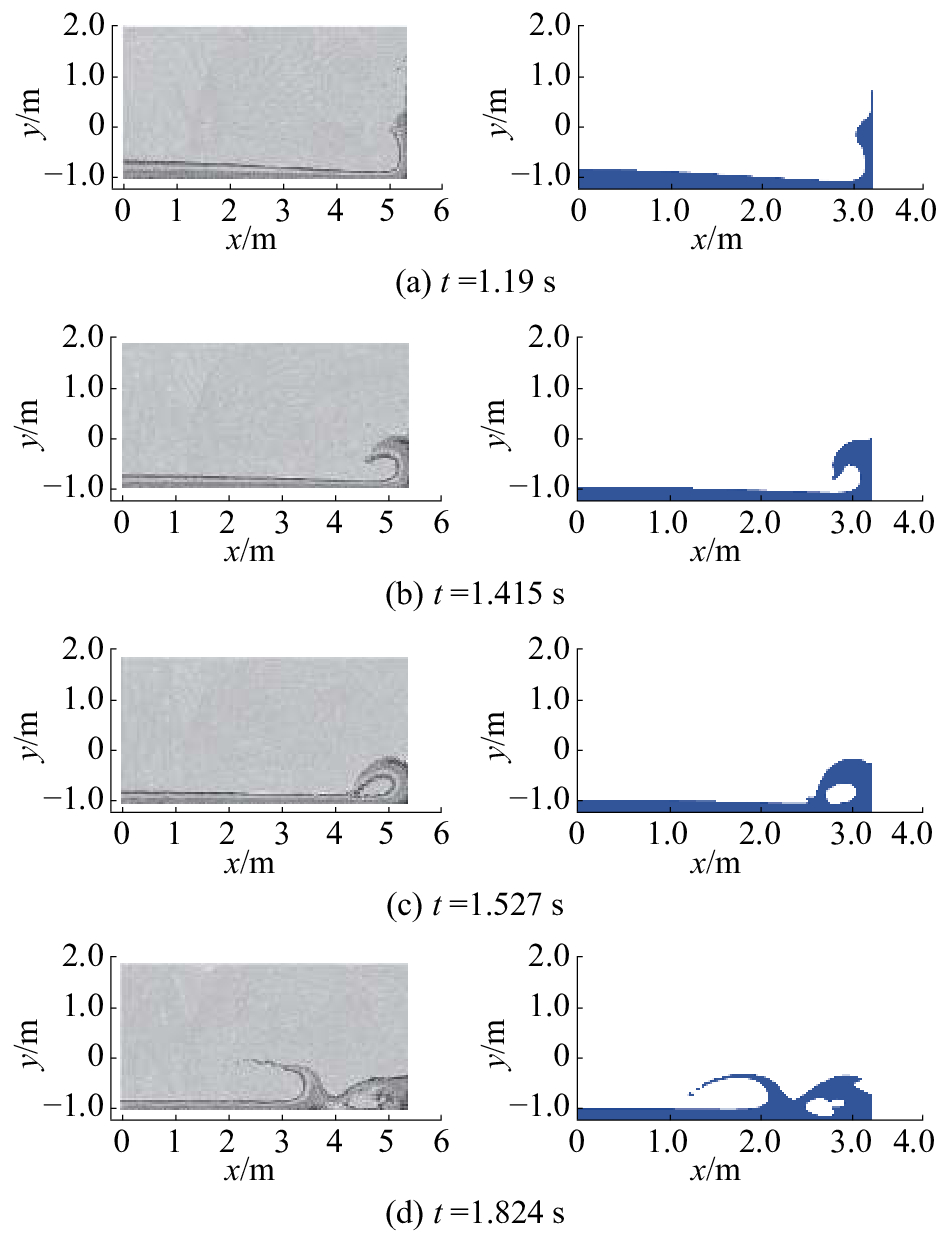Download: 图 4 自由液面演变与文献的对比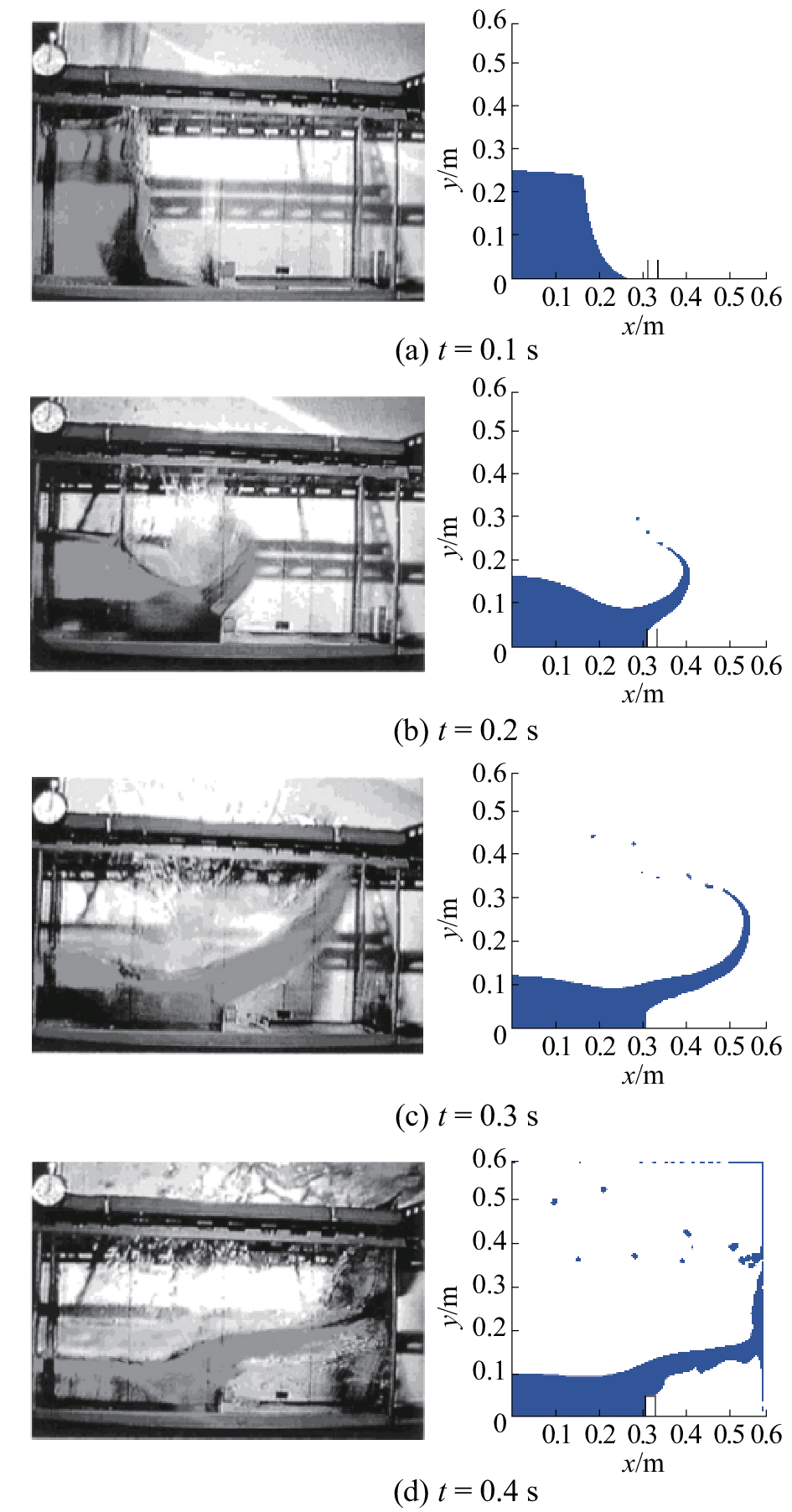Download: 图 5 带障碍物二维溃坝自由液面演变

4 结论

  GREAVES D M. Simulation of viscous water column collapse using adapting hierarchical grids[J]. International journal for numerical methods in fluids, 2006, 50(6): 693-711. DOI:10.1002/(ISSN)1097-0363 (0)  ABDOLMALEKI K, THIAGARAJAN K P, MORRIS-THMOAS M T. Simulation of the dam break peoblem and impact flows using a navier-stokes solver[C]//15th Australasian Fluid Mechanics Conference. Sydney, Australia, 2004. (0)  SINGH J, ALTINAKAR M S, DING Yan. Two-dimensional numerical modeling of dam-break flows over natural terrain using a central explicit scheme[J]. Advances in water resources, 2011, 34(10): 1366-1375. DOI:10.1016/j.advwatres.2011.07.007 (0)  BRUFAU P, GARCIA-NAVARRO P. Two-dimensional dam break flow simulation[J]. International journal for numerical methods in fluids, 2000, 33(1): 35-57. DOI:10.1002/(ISSN)1097-0363 (0)  陈朝晖. 固定方舱破损进水数值模拟[D]. 哈尔滨: 哈尔滨工程大学. 2015. (0)  孙东亮, 屈治国, 何雅玲, 陶文铨. 求解流动与传热问题的一种高效稳定的分离式算法—IDEAL[J]. 工程热物理学报, 2009, 30(08): 1369-1372. DOI:10.3321/j.issn:0253-231X.2009.08.031 (0)  SEIFOLLAHI M, SHIRANI E, ASHGRIZ N. An improved method for calculation of interface pressure force in PLIC-VOF methods[J]. European journal of mechanics-B/fluids, 2008, 27(1): 1-23. DOI:10.1016/j.euromechflu.2007.01.002 (0)  史宏达, 刘臻. 溃坝水流数值模拟研究进展[J]. 水科学进展, 2006(01): 129-135. DOI:10.3321/j.issn:1001-6791.2006.01.021 (0)  赵大勇, 李维仲. VOF方法中几种界面重构技术的比较[J]. 热科学与技术, 2003(04): 318-323. DOI:10.3969/j.issn.1671-8097.2003.04.008 (0)  王宗鹏. 基于有限体积法的液滴撞击固体平壁数值模拟[D]. 大连: 大连理工大学, 2010. (0)  MEIER M, YADIGAROGLU G, SMITH B L. A novel technique for including surface tension in PLIC-VOF methods[J]. European journal of mechanics-B/Fluids, 2002, 21(1): 61-73. DOI:10.1016/S0997-7546(01)01161-X (0)  MARKUS M. Numerical and experimental study of large steam-air bubbles injected in a water pool[D]. Zurich: ETH Zürich, 1999. (0)  GAO Zhiliang, VASSALOS D, GAO Qiuxin. Numerical simulation of water flooding into a damaged vessel’s compartment by the volume of fluid method[J]. Ocean engineering, 2010, 37(16): 1428-1442. DOI:10.1016/j.oceaneng.2010.07.010 (0)  HÄNSCH S, LUCAS D, HÖHNE T, et al. Application of a new concept for multi-scale interfacial structures to the dam-break case with an obstacle[J]. Nuclear engineering and design, 2014, 279: 171-181. DOI:10.1016/j.nucengdes.2014.02.006 (0)  陶文铨. 数值传热学[M]. 2版. 西安: 西安交通大学出版社, 2001: 244-245. (0)